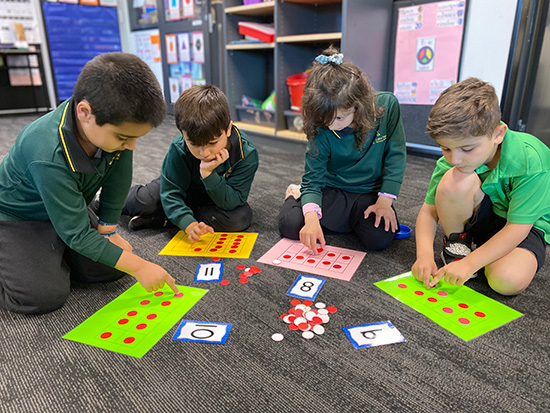# Numeracy### Mathematics teaches students how to make sense of the world around them through developing a child’s ability to calculate, reason and solve problems.

Mathematics is an essential area of learning in our curriculum. The importance of developing mathematical skills is vital for students to be able to reason, solve problems, demonstrate understanding and confidently navigate through life.

The mathematics program at Lalor North Primary School allows all students to have genuine access to high quality learning in mathematics. Students from Prep to Year 6 study Mathematics for at least five hours per week. Students will be encouraged to analyse, compare, explain, reason, justify, estimate, and synthesise mathematical problems, which will assist them in developing the ability to choose the most effective approach to solving problems.  Through the process of learning to be numerate, students recognise that mathematics is widely used both in and outside school and learn to apply mathematical knowledge and skills in a wide range of familiar and unfamiliar situations.

Students learn mathematics in the following strands;

• Number and Algebra
• Measurement and Geometry
• Statistics and Probability

Students will develop the following proficiencies;

• Understanding
• Fluency
• Reasoning
• Problem Solving